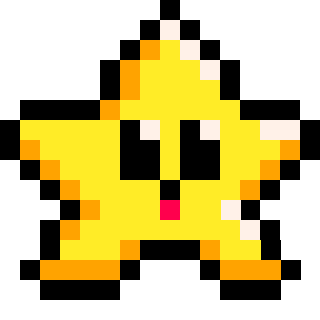# SUB

``sub( string, first, [last] )``
 string the string that you want to convert into a table first the number of characters from the beginning of the string to start pulling as the substring. *If not given, the full string is returned. last (optional) the number of characters from the beginning of the string to stop pulling as the substring. (default: full length of string)

The sub( string ) function is used to separate a part of a string into a smaller string. The first character of the string is 1.For example, if we call `sub("hello world")`, the function will return a character or string from within the full given string.

``````string = "hello world"
print( sub(string,1 ) )   --"hello world"
print( sub(string,2 ) )   --"ello world"
print( sub(string,4,8 ) ) --"lo wo"
print( sub(string,7,7 ) ) --"w"
``````### Common Uses

The most common usage of the function `sub()` is to create a text appearance animation as if it is being typed out one character at a time. Example:

``````string = "hello world"
first = 1
last = 1

function _draw()
cls()
last+=.5
print( sub(string,first,last) )
end``````

Another way to reveal text is to use the `clip()` function. See the tutorial Text Wipe for a detailed example.262

23 Nov 2023

`Font`